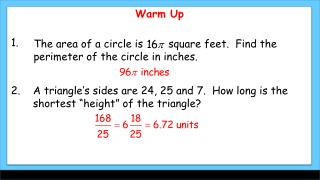Download PresentationWarm Up

# Warm Up - PowerPoint PPT PresentationDownload Presentation## Warm Up

- - - - - - - - - - - - - - - - - - - - - - - - - - - E N D - - - - - - - - - - - - - - - - - - - - - - - - - - -
##### Presentation Transcript

1. Warm Up 1. The area of a circle is square feet. Find the perimeter of the circle in inches. 2. A triangle’s sides are 24, 25 and 7. How long is the shortest “height” of the triangle?

2. Vocabulary Coordinate plane Leg Hypotenuse A coordinate planeis a plane that is divided into four regions by a horizontal line (x-axis) and a vertical line (y-axis) . The location, or coordinates, of a point are given by an ordered pair (x, y). Who is the “father” of the coordinate plane? Rene DesCartes

3. You can find the midpoint of a segment by using the coordinates of its endpoints. Calculate the average of the x-coordinates and the average of the y-coordinates of the endpoints. (-5, 5) Find the coordinates of the midpoint of PQ with endpoints P(–8, 3) and Q(–2, 7).

4. M is the midpoint ofAB. A has coordinates (2, 7) and M has coordinates (6, 1). Find the coordinates ofB.

5. What does the distance formula remind us of? Pythagorean Theorem

6. Find FG and JK. The segments are not congruent

7. I believe there are about 400 separate proofs of the Pythagorean theorem (including one written by a congressman who five years later became the President of the United States) … how times have changed.

8. Theorem Notebook – Write the first theorem in your theorem notebook. Include title (Theorem 1-6-1 Pythagorean Theorem), write out the theorem, and give illustration(s). Instead of writing your own proof, I want you to get on the internet and copy a proof that you can UNDERSTAND into your notebook. Pick the version you like best!

9. 1. Find the coordinates of the midpoint of MN with endpoints M(-2, 6) and N(8, 0). Lesson Quiz 2.K is the midpoint of segment HL. H has coordinates (1, –7), and K has coordinates (9, 3). Find the coordinates of L. 3. Find the exact distance between the points S(6, 5) and T(–3, –4). Mentallyestimateto nearest tenth. 4. The coordinates of the vertices of ∆ABC are A(2, 5), B(6, –2), and C(–4, –3). Without calculator, find the perimeter of ∆ABC to the nearest whole number.

10. Assignment today is page 47: 12-30 even, 33-41 and page 65: 1-5. Also, Pythagorean Theorem becomes the first entry in your theorem notebook. Remember that homework help is always available at http://www.thinkcentral.com/index.htm Today’s keyword is “MG7 1-6”.